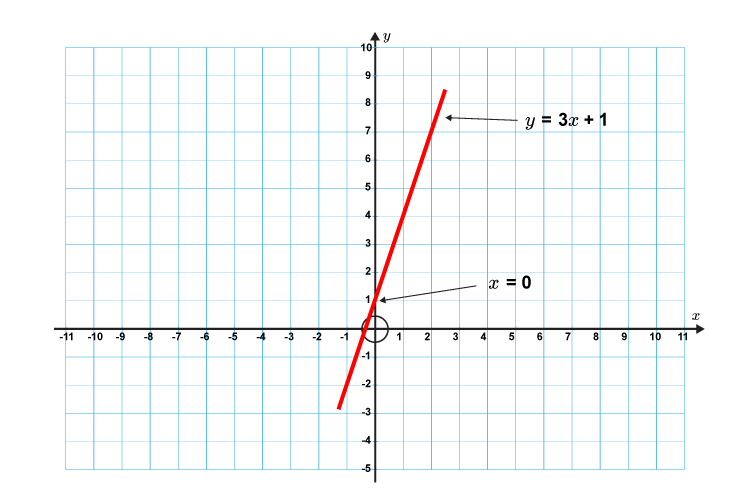# What does c represent

The formula of a straight line is y=mx+c

c is the point where the straight line crosses the y axis (y intercept).

## Do we need to remember this?

No because it is so easy to work outAs follows:

y=3x+1 is the formula of a straight line

If x is zero then:

y=3times0+1

y=1

The point where the slope hits the y axis is:

x=0 and y=1

Likewise

The point where the line hits the x axis is

y=3x+1

If y is zero then:

0=3x+1

3x=-1

x=-1/3

The point where the line hits the x axis is:

x=-1/3 and y=0